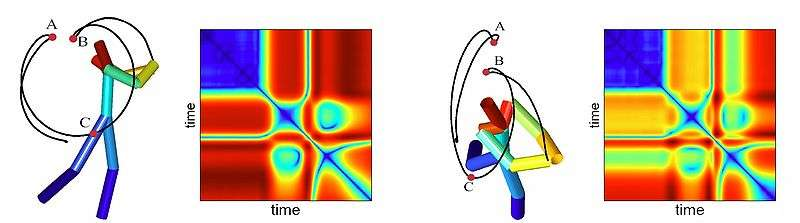# Self-similarity matrix

In data analysis, the self-similarity matrix is a graphical representation of similar sequences in a data series.

Similarity can be explained by different measures, like spatial distance (distance matrix), correlation, or comparison of local histograms or spectral properties (e.g. IXEGRAM). This technique is also applied for the search of a given pattern in a long data series as in gene matching. A similarity plot can be the starting point for dot plots or recurrence plots.

## Definition

To construct a self-similarity matrix, one first transforms a data series into an ordered sequences of feature vectors, where each vectordescribes the relevant features of a data series in a given local interval. Then the self-similarity matrix is formed by computing the similarity of pairs of feature vectorswhereis a function measuring the similarity of the two vectors, for instance, the inner product. Then similar segments of feature vectors will show up as path of high similarity along diagonals of the matrix. Similarity plots are used for action recognition that is invariant to point of view  and for audio segmentation using spectral clustering of the self-similarity matrix.

## ExampleSimilarity plot, a variant of recurrence plot, obtained for different views of human actions are shown to produce similar patterns.

1. M. A. Casey; A. Westner (July -00 2000). "Separation of mixed audio sources by independent subspace analysis" (PDF). Proc. Int. Comput. Music Conf. Retrieved 2013-11-19. Check date values in: |date= (help)